On the Tuning Parameter for the Adaptive Bonferroni Procedure under Positive Dependence | OMICS International
Journal of Biometrics & Biostatistics

Like us on:

# On the Tuning Parameter for the Adaptive Bonferroni Procedure under Positive Dependence

Ji Won Byun and Jianan Peng*

Department of Mathematics and Statistics, Acadia University, Wolfville, NS, B4P, 2R6, Canada

*Corresponding Author:
Jianan Peng
Department of Mathematics and Statistics
E-mail: [email protected]

Received Date: October 22, 2011; Accepted Date: January 21, 2012; Published Date: January 25, 2012

Citation: Byun JW, Peng J (2012) On the Tuning Parameter for the Adaptive Bonferroni Procedure under Positive Dependence. J Biomet Biostat 3:130. doi:10.4172/2155-6180.1000130

Copyright: © 2012 Byun JW, et al. This is an open-access article distributed under the terms of the Creative Commons Attribution License, which permits unrestricted use, distribution, and reproduction in any medium, provided the original author and source are credited.

Visit for more related articles at Journal of Biometrics & Biostatistics

#### Abstract

Guo introduced an adaptive Bonferroni procedure and he proved that his adaptive Bonferroni procedure controls the familywise error rate under a conditional dependence model. However, how to choose the tuning parameter λ to control the familywise error rate in the procedure under positive dependence is not clear in his paper. In this paper, we suggest that λ = α . Simulation studies are provided.

#### Keywords

Adaptive Bonferroni procedure; Familywise error rate; Holm procedure

#### Introduction

Simultaneously testing a family of m null hypotheses Hi(i =1,...,m) can arise from many circumstances such as comparing several treatments with a control. A main concern in multiple testing is the multiplicity problem, namely, that the probability of committing at least one Type I error sharply increases with the number of the hypotheses tested at a prespecified level. The probability of at least one false rejection is referred to as the familywise error rate (FWER). Several procedures have been proposed for controlling the familywise error rate, including proposals by Holm  and Hochberg . When some null hypotheses are false, these procedures are often conservative by a factor given by the proportion of the true null hypotheses among all null hypotheses.By exploiting knowledge of this proportion Hochberg & Benjamini  introduced adaptive Bonferroni, Holm and Hochberg procedures for controlling the familywise error rate. These adaptive procedures estimate, the proportion and then use it to derive more powerful testing procedures. However, whether or not the adaptive procedure ultimately control the FWER has not yet been mathematically established. Recently, Guo  offered a partial answer to the open problem. He considered the aforementioned adaptive Bonferroni procedure, modified it slightly by replacing the estimate of the number of true null hypothesis by the estimate that Storey et al.  considered in the context of false discovery rate, and proved that, when the p-values are independent or exhibit certain types of dependence, his version of adaptive Bonferroni procedure controls the FWER. Guo  conducted a simulation study for positive correlated p-values with the tuning parameter λ = 0.2 to show his procedure controlling FWER. However, Finner and Gontscharuk  reported that the adaptive Bonferroni procedure does not control FWER when λ = 0.5 for positive highly-correlated p-values. Guo  did not explain why he chose the tuning parameter λ = 0.2. These motivated us to do a further simulation study for the adaptive Bonferroni procedure. In this paper we propose to use λ = α. Then Guo’s adaptive procedure controls FWER for positive correlated p-values. This observation has not been reported in the literature.

Given m null hypotheses H1 ,...,Hm, consider testing if Hi = 0, true, or Hi = 1, false, simultaneously for i =1,...,m, based on their respective p–values P1,..,Pm. Assume that Hi(i=1,...,m), are Bernoulli random variables with pr(H=0)=π0 =1−pr(H=1), and the corresponding p– values Pi can be expressed as

Pi = (1 - Hi )Ui + HiG−1(Ui),                                                                   (2.1)

where Ui(i=1,...,m) are independent and identically distributed uniform (0,1) random variables that are independent of all Hi;Gi is some cumulative distribution function on (0,1) and Gi−1(u) is the inverse of Gi. The Pis are conditionally independent given Hi(i=1,...,m), but His may be dependent. If the His are independent, then (2.1) reduces to the conventional random effect model [7-9].

If V is the number of true null hypotheses rejected, then the familywise error rate is defined to be the probability of one or more false rejections, i.e. FWER = pr{V > 0}. Let P1:m ≤···≤ Pm:m be the ordered values of P1,...,Pm and H(1),...,H(m) be the corresponding null hypotheses. The Bonferroni procedure controls the familywise error rate at level π0α for test statistics with arbitrary dependence by rejecting Hi whenever Pi≤ α/m. Holm  proposed a step-down version of the Bonferroni procedure, which controls the familywise error rate at α. Let αi = α/(m− i+1)(i = 1,...,m) and r be the largest i such that P1:m ≤ α1,...,Pi:m ≤ αi, then under the Holm procedure, we reject the hypotheses H(1),...,H(r). If r is not defined, then no hypothesis is rejected.

Because the above Bonferroni-type procedures are conservative by the factor π0, knowledge of π0 can be useful for improving the performance of Bonferroni and Holm’s procedures. Several estimators of π0 have been introduced; see [5,10], among others. Guo  used Storey et al.’s simple estimator: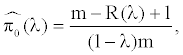(2.2)

where 0 < λ < 1 is a prespecified constant,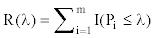is the number of p-values less than or equal to λ, and I( ) is an indicator function. Storey et al.’s estimator is a simplified version of Schweder and Spjotvoll’s estimator, which was used in the adaptive procedures of Hochberg & Benjamini  and Benjamini & Hochberg . Based on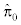(λ ) , Guo’s adaptive Bonferroni procedure is defined as follows

Definition 2.1 The level α adaptive Bonferroni procedure.

1. Given a fixed λ∈(0,1), findand then calculatebased on (2.2).

2. Reject H(1),...,H(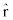) , where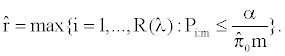If the maximum does not exist, reject no hypothesis. Guo proved that the adaptive Bonferroni procedure above controls the familywise error rate at level α in the conditional dependence model.

#### Simulations of Familywise Error Rate and Power for Dependent p-values

It is recognized that the dependence issue is always very complicated in multiple testing. We simulate six different types of dependence structures to compare numerically the FWER control level and the power of Guo’s adaptive Bonferroni procedure (denoted by a Bon in tables) with that of the Bonferroni (denoted by Bon in tables) and Holm procedures for dependent p-values. We set α = 0.01, 0.05 and λ= α, 0.1, 0.2, 0.5 depending on the type of dependence structure of p-values. The simulated FWER and average power, the expected proportion of false nulls that are rejected, are based on 10000 replications. With 10000 repetition, the standard error of the estimated coverage near α is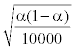, and it never exceeds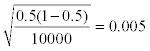.

Example 1 (positive equicorrelation)

In this example, our simulation study is similar to Guo’s simulation study. The number of tests m = 200 was set for H0i : μi=0 against Hai : μi ≠ 0 with the fraction of the true null hypotheses π0 = 0.1,0.2,...,0.9. Let Z0,Z1,...,Zm be distributed independently and identically as N(0,1) and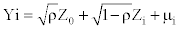, where ρ = 0.1,...,0.9 and μi = 0,i = 1,...,m0 = π0m, μi = 6, i = m0+1,...,m. We only report ρ = 0.5,0.9 here for space limits. When ρ = 0,Yi are independent and the p-values are independent, a special case of the conditional dependence model. Guo studied λ=0.2 for α = 0.05 and 1000 replications. Note that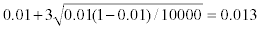When α = 0.01 and λ = 0.2, Tables 1 and 2 indicate that the adaptive Bonferroni procedure does not control FWER for ρ = 0.5, 0.9; when ρ = 0.9, the adaptive Bonferroni procedure does control FWER even for λ = 0.1. Table 3 indicates that for λ = 0.2 the adaptive Bonferroni procedure controls FWER when α = 0.05 and ρ = 0.5, which matches the result in Guo . Table 4 demonstrates that when π0 = 0:1; 0:2, the adaptive Bonferroni procedure does not control FWER for λ = 0.2 when α = 0.5 and ρ = 0.9 (note that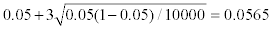). However, for λ = α, the adaptive Bonferroni procedure does control FWER for all ρ =0.1,...,0.9 and its FWER level is more closer to α than the Bonferroni procedure and the Holm procedure. The powers of the adaptive Bonferroni procedure are larger than the powers of the Bonferroni procedure and the Holm procedure even for λ = α from Tables 1-4.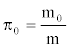Bon Holm adaptive Bon λ=0.2 λ=0.1 λ=α 0.1 .0006(.9743) .0059(.9911) .0167(.9960) .0127(.9953) .0081(.9940) 0.2 .0016(.9743) .0053(.9879) .0157(.9930) .0121(.9919) .0077(.9903) 0.3 .0024(.9742) .0065(.9854) .0160(.9905) .0126(.9892) .0079(.9875) 0.4 .0034(.9741) .0067(.9832) .0154(.9882) .0128(.9868) .0085(.9849) 0.5 .0042(.9741) .0067(.9813) .0152(.9861) .0127(.9847) .0085(.9827) 0.6 .0048(.9741) .0068(.9796) .0153(.9844) .0126(.9828) .0082(.9808) 0.7 .0054(.9740) .0070(.9779) .0155(.9826) .0119(.9810) .0084(.9789) 0.8 .0060(.9739) .0072(.9764) .0156(.9811) .0121(.9793) .0081(.9772) 0.9 .0069(.9740) .0076(.9752) .0158(.9796) .0126(.9777) .0089(.9757)

Table 1: FWER and power when α =0.01, ρ =0.5.

<Bon Holm adaptive Bon λ=0.2 λ=0.1 λ=α 0.1 .0003(.9735) .0014(.9853) .0292(.9997) .0172(.9996) .0043(.9948) 0.2 .0004(.9734) .0014(.9834) .0327(.9997) .0208(.9994) .0047(.9910) 0.3 .0005(.9735) .0013(.9818) .0341(.9996) .0197(.9991) .0040(.9879) 0.4 .0007(.9734) .0010(.9802) .0338(.9996) .0191(.9985) .0040(.9850) 0.5 .0008(.9734) .0011(.9789) .0335(.9995) .0191(.9979) .0036(.9828) 0.6 .0009(.9734) .0010(.9776) .0333(.9994) .0188(.9973) .0032(.9806) 0.7 .0009(.9734) .0010(.9765) .0327(.9993) .0182(.9965) .0031(.9787) 0.8 .0009(.9733) .0010(.9754) .0323(.9992) .0175(.9958) .0032(.9770) 0.9 .0009(.9732) .0010(.9743) .0318(.9991) .0176(.9950) .0030(.9753)

Table 2: FWER and power when α = 0.01, ρ = 0.9.Bon Holm adaptive Bon λ=0.2 λ=α 0.1 .0037(.9904) .0345(.9979) .0539(.9993) .0434(.9988) 0.2 .0072(.9903) .0284(.9967) .0475(.9985) .0368(.9978) 0.3 .0108(.9903) .0279(.9956) .0465(.9977) .0343(.9968) 0.4 .0133(.9903) .0263(.9947) .0439(.9970) .0315(.9959) 0.5 .0158(.9903) .0259(.9938) .0423(.9963) .0320(.9951) 0.6 .0178(.9903) .0257(.9931) .0429(.9956) .0312(.9942) 0.7 .0203(.9903) .0253(.9923) .0414(.9950) .0308(.9935) 0.8 .0224(.9902) .0258(.9916) .0404(.9944) .0313(.9928) 0.9 .0241(.9903) .0262(.9910) .0408(.9940) .0311(.9922)

Table 3: FWER and power when α = 0.05, ρ = 0.5.Bon Holm adaptive Bon λ=0.2 λ=α 0.1 .0017(.9899) .0107(.9956) .0636(1.0000) .0268(.9999) 0.2 .0022(.9899) .0074(.9948) .0585(1.0000) .0228(.9999) 0.3 .0023(.9899) .0069(.9939) .0549(.1.0000) .0211(.9998) 0.4 .0025(.9899) .0058(.9931) .0528(1.0000) .0196(.9997) 0.5 .0027(.9899) .0054(.9925) .0504(1.0000) .0192(.9996) 0.6 .0028(.9899) .0044(.9919) .0484(1.0000) .0182(.9995) 0.7 .0029(.9899) .0042(.9914) .0481(1.0000) .0176(.9993) 0.8 .0033(.9900) .0042(.9910) .0471(1.0000) .0168(.9992) 0.9 .0036(.9900) .0043(.9905) .0471(1.0000) .0165(.9990)

Table 4: FWER and power when α = 0.05, ρ = 0.9.

Example 2 (positive block dependence)

This example largely follows the set-up of Example 3 in Finner and Gontscharuk . Let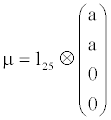and Σ = σ2 J25 ⊗ {(1-ρ) J4+ρ14×4}, ρ ∈ (0,1), where 1n denotes a column vector of 1s of length n,1n×n denotes an n×n matrix of 1s and Jn is the identity matrix. Let Xj ~N100(μ,Σ), j = 1,...,n, be independent and identically distributed. We use σ =1 and a =1.0,1.5,2.0 in the simulation. We consider the multiple test problem Hai : μi = 0 versus Hai : μi ≠ 0, i = 1,..,100. We use the test statistic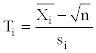Gontscharuk , where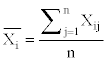and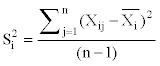Therefore, the test statistics have a t(n-1) distribution. The p-values corresponding to Ti is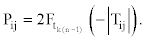,where Ftv denotes the cumulative distribution function of a central t-distribution with v degrees of freedom. For illustration, we simulate this model for n = 10, a = 2.0; n = 16, a = 1.5; n = 25, a = 1.0, and only three values of ρ = 0.1,0.5,0.9. Table 5 indicates that the adaptive Bonferroni procedure controls FWER well for each ρ and λ. Moreover, for the adaptive Bonferroni procedure, FWER decreases slightly but power does not change much when ρ increases and its FWER and power seem to be nearly independent of λ.

 r Procedure n=10, a=2.0 n=16, a=1.5 n=25, a=1.0 FWER power FWER power FWER power 0.1 Bon .0257 .7740 .0269 .9015 .0272 .8115 aBon(λ=0.5) .0500 .8637 .0497 .9433 .0505 .8699 aBon(λ=0.2) .0504 .8648 .0494 .9438 .0499 .8708 aBon(λ=0.05) .0498 .8651 .0503 .9440 .0497 .8708 Holm .0442 .8491 .0477 .9411 .0451 .8608 0.5 Bon .0224 .7741 .0261 .9012 .0267 .8119 aBon(λ=0.5) .0492 .8644 .0472 .9431 .0499 .8697 aBon(λ=0.2) .0476 .8659 .0473 .9435 .0499 .8706 aBon(λ=0.05) .0481 .8662 .0471 .9436 .0488 .8706 Holm .0418 .8497 .0444 .9408 .0430 .8608 0.9 Bon .0216 .7745 .0223 .9012 .0208 .8123 aBon(λ=0.5) .0461 .8646 .0422 .9425 .0421 .8708 aBon(λ=0.2) .0467 .8659 .0416 .9437 .0424 .8711 aBon(λ=0.05) .0458 .8663 .0411 .9437 .0418 .8710 Holm .0394 .8496 .0388 .9406 .0360 .8610

Table 5: Simulation study for the positive block dependence model in example2 for α = 0.05.

Example 3 (pairwise comparisons)

This example is modified from Example 2 in Finner and Gontscharuk . Let Xij, i = 1,..,k,j=1,..,n, be independent normally distributed random variables with unknown mean μi and unknown variance σ2. We consider the pairwsie comparisons problem

Hoij : μij versus Haij : μi ≠ μj,1 ≤ i < j ≤ k

for various scenarios of means. The test statistics are given by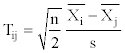withand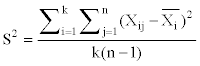Therefore, the test statistics have a tk(n-1) distribution. The p-values corresponding to Tij isSetting t0=0, a scenario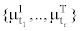means thatfor i = 1,..,r. So the case μ1 = μ2 = μ3 = 0, μ4 = μ5 = μ6 = μ7= 2 and μ8 = μ9 = μ10 = 4 corresponds to {03, 24, 43} with k =10 and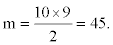Table 6 shows that the adaptive Bonferroni procedure apparently controls FWER for all λ and it is more powerful than the Bonferroni procedure and the Holm procedure.

 μ - scenario Procedure Results for n = 4 Results for n = 6 Results for n = 8 FWER Power FWER Power FWER Power {03,24,43}, Bon .0107 .4442 .0120 .6420 .0118 .7967 m=45,m0 =12 aBon(λ=0.5) .0450 .5471 .0505 .7488 .0530 .8788 aBon(λ=0.2) .0381 .5428 .0445 .7505 .0504 .8804 aBon(λ=0.05) .0298 .5261 .0369 .7442 .0434 .8792 Holm .0211 .4825 .0263 .7070 .0328 .8628 {04,11,24,31}, Bon .0125 .2250 .0131 .3963 .0145 .5250 m=45, m0 =12 aBon(λ=0.5) .0350 .2949 .0382 .4776 .0444 .6016 aBon(λ=0.2) .0313 .2859 .0329 .4706 .0379 .5966 aBon(λ=0.05) .0239 .2702 .0263 .4565 .0306 .5853 Holm .0173 .2409 .0192 .4261 .0219 .5609 {04,14,24,34,44}, Bon .0071 .2760 .0077 .4078 .0071 .4954 m=190, m0 =30 aBon(λ=0.5) .0265 .3366 .0300 .4748 .0327 .5643 aBon(λ=0.2) .0221 3275 .0259 .4660 .0287 .5564 aBon(λ=0.05) .0161 .3143 .0198 .4532 .0214 .5439 Holm .0108 .2899 .0113 .4288 .0131 .5206

Table 6: Simulation study for the pairwise comparisons problem in example 3 for α=0.05.

Example 4 (Storey et al. ’s block dependence)

This example follows Storey, Taylor and Siegmund ’s dependence example. The null statistics have N(0,1) marginal distribution with m0 = 60,240 and the alternative distributions have marginal distribution N(6,1) with m1= m - m0 = 240,60 respectively. The statistics have correlation ± ρ = 0.1,..,0.9 in group size of 10 as the following.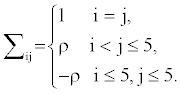See Storey et al.  for details. FWER is well controlled for all the procedures and λ choices. For brevity, Table 7 lists the results for ρ = 0.1,0.5,0.9 only.Procedure Results for ρ=0.1 Results for ρ=0.5 Results for ρ=0.9 FWER power FWER power FWER power 0.2 Bon .0118 .9873 .0106 .9874 .0097 .9873 aBon(λ=0.5) .0492 .9960 .0485 .9960 .0411 .9961 aBon(λ=0.2) .0486 .9960 .0487 .9960 .0412 .9961 aBon(λ=0.05) .0489 .9960 .0482 .9960 .0406 .9961 Holm .0483 .9960 .0477 .9960 .0380 .9959 0.8 Bon .0383 .9872 .0366 .9873 .0224 .9874 aBon(λ=0.5) .0480 .9890 .0466 .9892 .0288 .9894 aBon(λ=0.2) .0483 .9890 .0470 .9892 .0279 .9894 aBon(λ=0.05) .0486 .9890 .0460 .9891 .0277 .9893 Holm .0480 .9890 .0455 .9891 .0270 .9891

Table 7: Simulation study for the block dependence model in example 4 for α=0.05.

Example 5 (negative block dependence)

The set-up is similar to Example 4 above but the statistic correlation is negative. The null statistics have N(0,1) marginal distribution with m0 = 320 and the alternative distributions have marginal distribution N(0,6) with m1 = m - m0 = 80. The statistics have correlation -ρ in group size of 2 as the following.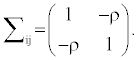FWER is well controlled for all the procedures and λ choices. For brevity, Table 8 lists the results for correlation -0.1,-0.5,-0.9 only.

 Procedure Results for m0 =80 Results for m0 =160 Results for m0 =190 FWER Power FWER Power FWER Power Bon .0381 .9845 .0369 .9846 .0322 .9846 aBon(λ=0.5) .0485 .9866 .0454 .9866 .0383 .9866 aBon(λ=0.2) .0481 .9866 .0454 .9866 .0384 .9866 aBon(λ=0.05) .0480 .9866 .0457 .9866 .0385 .9866 Holm .0482 .9865 .0456 .9866 .0387 .9866

Table 8: Simulation study for the negative block dependence model in example 5 for a = 0.05.

Example 6 (multivariate equicorrelated t-distribution)

We consider the situation that was described in Example 1 and Example 5 in Finner and Gontscharuk . Let Let Xi ~N(μi2), i = 1,...,m be independent normal random variables and let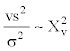be independent of the Xis. The multiple testing problem is H0i : μi = 0 versus Hai : μi > 0,i = 1,...,m with test statistic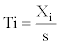Then T = (T1,...,Tm) has a multivariate equicorrelated t-distribution. The p-values are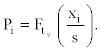In the simulation, we have m = 200, ν = 15, σ2 = 1, μi= 0, i = 1,..., m0, and μi = 6, i = m0 +1,..., m, m0 = 80, 160, 190. Table 9 demonstrates that FWER is obviously controlled for all values of m0 and λ that are considered in the simulation. Moreover, the differences between the three procedures in FWER and power are virtually negligible and independent of the choice of λ when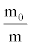is large.

 Procedure Results for m0 =80 Results for m0 =160 Results for m0 =190 FWER Power FWER Power FWER Power Bon .0158 .8981 .0279 .8984 .0320 .8984 aBon(λ=0.5) .0375 .9524 .0341 .9137 .0331 .9016 aBon(λ=0.2) .0413 .9502 .0352 .9103 .0367 .8980 aBon(λ=0.05) .0407 .9515 .0346 .9119 .0349 .8998 Holm .0375 .9423 .0332 .9104 .0336 .9011

Table 9: Simulation study for the multivariate equicorrelated t-distribution model in example6 for α = 0.05.

#### Discussion

Guo  mathematically proved that the adaptive Bonferroni procedure controls FWER under a conditional dependence model. A critical point for the adaptive Bonfer-roni procedure is the choice of the tuning parameter λ. Finner and Gontscharuk  suggested that λ around ½ may be a good compromise and they further commented that \Anyhow, it seems not easy to give precise guidelines here” (page 1046 of their paper). It is a challenging problem for the proof of FWER control for the adaptive Bonferroni procedure under dependent p-values. In this paper, we suggest that λ = α as a guideline, it seems that the adaptive Bonferroni procedure controls FWER for the positve equicorrelated normal distributions in our simulations. This simple choice for the tuning parameter λ will help applications of Guo’s adapative Bonferroni procedure.

#### Acknowledgements

This work was supported in part by the Natural Sciences and Engineering Research Council of Canada and the Acadia University Research Fund for Peng. The authors would like to thank the anonymous reviewer for his/her insightful and useful comments, which helped to improve an earlier version of the article.

#### References

Select your language of interest to view the total content in your interested language

### Article Usage

• Total views: 12080
• [From(publication date):
January-2012 - Dec 15, 2019]
• Breakdown by view type
• HTML page views : 8296Can't read the image? click here to refresh